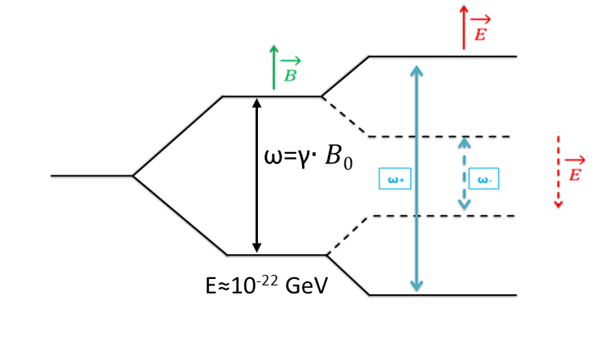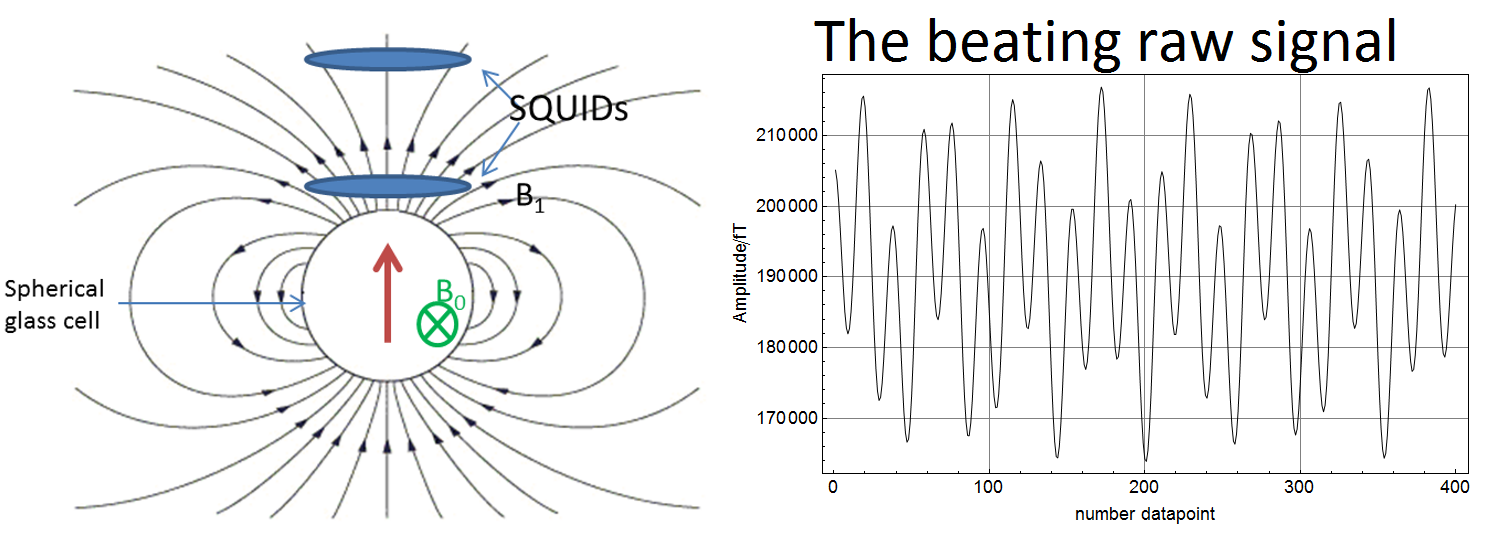# Introduction

## Introduction

The most precise tests of new physics are often performed in experiments that compare the transition frequencies of two co-located systems, typically radiating on their Zeeman or hyperfine transitions. For example, one could use a mixture of nuclear spin hyperpolarized 3He and 129Xe and compare their Larmor frequencies (wHe = gHeB0) and detect frequency changes due to an additional interaction. An essential assumption in this so-called clock comparison experiments is that the additionalinteraction does not couple to magnetic moments but directly to the sample spins. This direct coupling allows co-magnetometry that uses two different spin species to distinguish between a normal magnetic field and an anomalous field coupling. The advantage of differential measurements is that they are insensitive to common systematic effects, such as uniform magnetic field fluctuations. This gives us the possibility to search for new fundamental interactions, such as:

1. the interaction of spins with a background field which implicates violation of the Lorentz symmetry;
2. the short-range interaction of spins with unpolarized matter (search for CP-violating interactions, mediated by light, pseudoscalar bosons like the Axon);
3. the interaction of spins with the electric dipole moment of the 129Xe atom.
These non-magnetic interactions of the spins generate an energy-splitting, respectively a frequency shift Dw = Vnon-magn/ℏ similar to the magnetic interaction.Theoretically one spin species (e.g. 129Xe) would be sufficient to detect small changes of the transition frequency due to an additional interaction,provided that the magnetic guiding field B0 isconstant during the measurement. As the field drifts are about 1pT per hour the magnetic guiding field B0 is not constant and therefor the variation of the Larmorfrequency is much bigger than the small frequency (~nHz) shifts we are looking for.That is why we are using a 3He-129Xe-Comagnetometer. Both gases are hyperpolarized by optical pumping and filled into a glass cell. After excitation, the spins start precessing around the direction of a static magnetic field B0. The frequencies of the spin precession signals are measured by LTc-SQUID detectors. If we compose the frequency difference, whereby the frequency of Xenon is weighted with the gamma ratios of the two gases, the dependence on magnetic field fluctuations should drop out, and we get sensitive to the frequency shifts due to non-magnetic spin interaction:

Dw = wHe - gHe/gXe · wXe

The essential difference, in particular for instance to the 3He/129Xe spin masers used so far, is, that by monitoring the free spin precession, an ultra-high sensitivity can be achieved with a clock which is almost completely decoupled from the environment.The signal of the precessing spins decays exponentially with the so called transverse relaxation time T2. To determine the frequency of this signal with a high accuracy we need a long spin coherence time, respectively a long T2-time. Therefore we need a magnetic guiding field B0 with small field gradients (on the picotesla per cm scale).